Courses

# Poly Phase AC Circuit - 2

## 15 Questions MCQ Test Topicwise Question Bank for Electrical Engineering | Poly Phase AC Circuit - 2

Description
This mock test of Poly Phase AC Circuit - 2 for Electrical Engineering (EE) helps you for every Electrical Engineering (EE) entrance exam. This contains 15 Multiple Choice Questions for Electrical Engineering (EE) Poly Phase AC Circuit - 2 (mcq) to study with solutions a complete question bank. The solved questions answers in this Poly Phase AC Circuit - 2 quiz give you a good mix of easy questions and tough questions. Electrical Engineering (EE) students definitely take this Poly Phase AC Circuit - 2 exercise for a better result in the exam. You can find other Poly Phase AC Circuit - 2 extra questions, long questions & short questions for Electrical Engineering (EE) on EduRev as well by searching above.
QUESTION: 1

### Three equal resistances connected in star take a line current of 10 A when fed from 400 V, 50 Hz source. If the load resistances are reconnected in delta, the line current would be

Solution: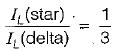or,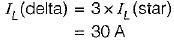QUESTION: 2

Solution:
QUESTION: 3

### The input to the 3-phase, 50 Hz circuit shown in figure below is 100 V. For a phase sequence of ABC, the wattmeters would read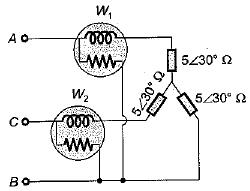Solution:

Wattmeter readings are:
W1 = VLIL cos (30 + φ)
and W2 = \/LIL cos (30 - φ)
Here,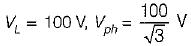and φ = 30°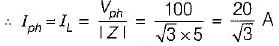Now,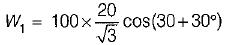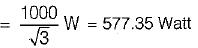and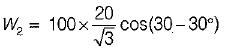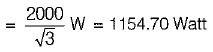QUESTION: 4

Three-phase power can be measured by two-wattmeter method in case of

Solution:
QUESTION: 5

In the case of power measured by two-wattmeter method in a balanced 3-phase system with a pure capacitive load

Solution:

W1 = VIL cos (30° + φ)
and W= VIL cos(30° - φ)
When the load is purely capacitance, then φ = - 90°.
So, W1 = VLI cos(30°- 90°)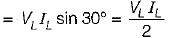and W2 = VLIL cos (30° + 90°)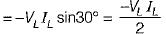Hence, |W1| = |W2| but W1 = - W2

QUESTION: 6

Measurement of power factor of a 3-phase system by two-wattmeter method can be obtained in case of

Solution:
QUESTION: 7

Two wattmeters used to measure power of a 3-phase balanced load reads W1, and W2. The reactive Dower drawn bv the load is

Solution:

We know that:
(W- W2) = VLILsin φ = Q1 - φ
So, Q3 - φ = √3(W1 - W2)

QUESTION: 8

Two wattmeters used to measure power of a 3-phase balanced load reads W1 and W2. The' reactive Dower drawn bv the load is

Solution:

We know that:
(W1 - W2) = VL IL sin φ = Q1-φ
so, Q3 - φ = √3(W1 - W2)

QUESTION: 9

A three-phase 220 V supply is applied to a balanced Δ-connected three phase load. The phase current being Iab =. 10∠-30°A as shown in the given figure, The total power received by the Δ-load is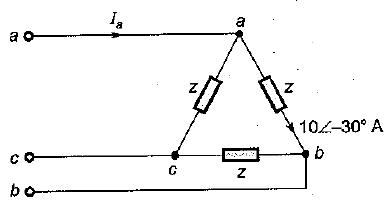Solution:

Iab = 10∠-30°A
So, Ibc = 10∠-150°A
and Ica = 10∠-270° A
Phase current,
Ia = Iab - Ica = (10∠-30° - 10∠-270°) A
= 10[cos 30° - jsin 30° - cos 270° + jsin 270°]
= 10[0.866 - j0.5 - 0 - j1]
= 8.66 - j15 = 17.32 ∠-60° A
∴ Ia = 17.32 ∠-60°A
∴ Total power received
= √3 VLIL cos φL
= 3VPIP cos φph
= 3 x 220 x 10 x cos 30°
= 5715W

QUESTION: 10

The magnitude of line current in the circuit shown below is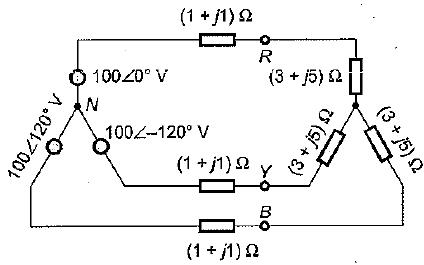Solution:

Impedance per phase
= 1 + j1 + 3 + j5
= (4 + j6) Ω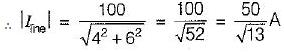QUESTION: 11

A three-phase, 400 V ac system is connected across a delta connected load having load resistance per phase of 200 Ω. If one of the phases of the delta connected load experiences an open circuit, then power consumed by the load will be

Solution:

Initially, in closed - Δ, power consumed by the load is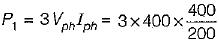= 2400W
Let, P2 be the power consumed in open delta.
then,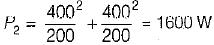QUESTION: 12

Assertion (A): Generated voltage or current wave have no odd harmonics.
Reason (R): The field system and armature coils of the generators are all symmetrical and so generates mostly symmetrical voltage wave.

Solution:
QUESTION: 13

Assertion (A): RMS value of line voltage is more than √3 times of the rms value of phase voltage.
Reason (R): In a star-connected system, the line voltage contains no triple frequency harmonic content.

Solution:
QUESTION: 14

Assertion (A): In a balanced 3-phase star connected system, line voltages are 30° ahead of the respective phase voltages.
Reason (R): The line voltages are √3 times of the respective phase voltages,

Solution:
QUESTION: 15

Match the List - I (Power factor) with List - II (Wattmeter readings) for the measurement of power in a three-phase system using two-wattmeter method and select the correct answer using the codes given below the lists:
List-I
A. 0.5 lag
B. Unity
C. Zero
D. 0.866

List-II
1. W1 = W2
2. W1 = 2W2
3. W1 > 0,  W2 = 0
4. W1 > 0, W2 < 0

Codes: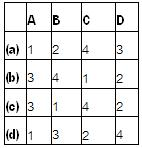Solution:

W1 = VLIL cos (30 + φ)
and W2 = VLIL cos (30 - φ)
By finding φ from cos φ, we can find the values of W1 and W2.